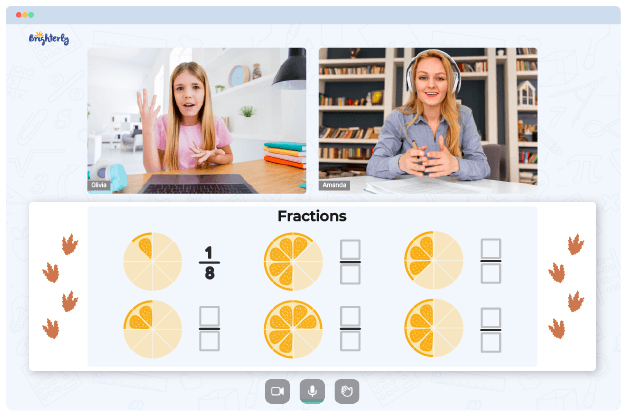# Equivalent Fractions Worksheet 4th Grade

Equivalent fractions can be challenging to digest. Students should identify the value fractions equal to and simplify them using addition, subtraction, and multiplication skills, as well as fundamental knowledge of fractions.

If your child feels uneasy about equivalent fractions, you can use extra learning materials – equivalent fraction worksheets for grade 4 from Brighterly. These studying materials contain simple and fun exercises with explanatory visuals to help children understand this topic better and simplify fractions correctly.

## 3 Benefits of Using a 4th-Grade Equivalent Fractions Worksheet

Equivalent fractions worksheets offer three advantages to students:

### Equivalent Fractions Worksheets for 4th-Grade Develop Fluency in Fractions

Brighterly’s equivalent fractions worksheets are precise learning tools that explain all ins and outs of this topic to children.

Fourth-graders will get extra practice hours to work on interactive drills that explain the topic from different angles and promote logical thinking.

Math for Kids

Is Your Child Struggling With Math?
1:1 Online Math Tutoring### Equivalent Fractions Worksheet for the 4th Grade Keep Children Interested in the Topic

A few children like formulas as they are. Kids may lose interest in something that has no entertainment aspect but requires increased mental effort. Using colorful worksheets with games and puzzles will help you keep your child interested in the topic and improve their focus.

### Equivalent Fractions 4th-Grade Worksheets Improve Number Sense and Math Skills

To solve equivalent fractions, you have to calculate numbers in your head. You have to cross multiply denominators or divide the bigger fraction by the value you already see it will work with.### Equivalent Fractions Worksheet 4th Grade PDF### Equivalent Fractions Worksheet 4th Grade PDF

Equivalent Fractions Worksheet 4th Grade PDF### Equivalent Fractions Worksheet 4th Grade PDF### Equivalent Fractions Worksheet 4th Grade PDF

Equivalent Fractions Worksheets For 4th Grade

Either way, you have to rely on previous math concepts – multiplication, addition, subtraction, and division. Working on equivalent fractions worksheets is an excellent chance to strengthen acquired math skills and develop numerical fluency.

## Get an Equivalent Fractions Worksheet 4th-Grade in PDF at Brighterly

Helping your kid with online math lessons for 4th grade. Expert tutors inspiring kids to love math so they can excel at It.

### Fractions Worksheets

Need help with Fractions?• Start lessons with an online tutor.

Is your child finding it challenging to grasp fractions concepts? An online tutor could be the answer.